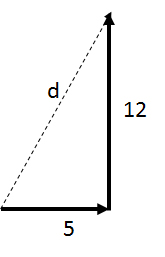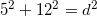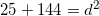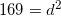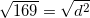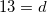# GED Science : Displacement

## Example Questions

### Example Question #1 : Displacement

A car drivesmiles east andmiles north. What is the total displacement of the car?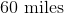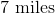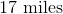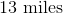Explanation:

The car has covered a total distance ofmiles, however, displacement and distance are not the same thing. Distance is simply the measure of how far the car have moved, regardless of direction. Displacement is a vector quantity, and takes direction into account. Displacement measures the total distance only between the car's starting point and ending point.

To solve this question, it is best to draw a sketch. The resulting sketch will be a right triangle with the hypotenuse equal to the displacement. The Pythagorean Theorem can be used to solve the problem.